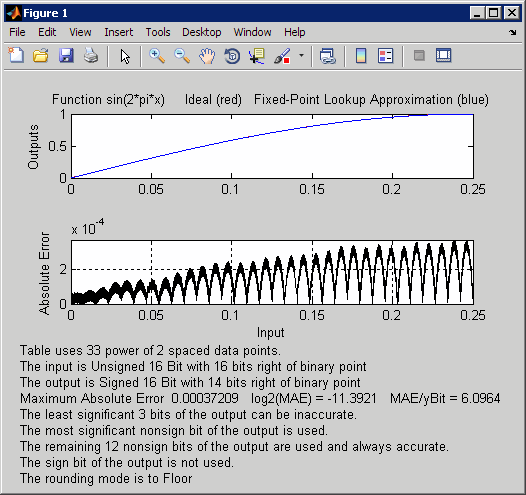# fixpt_look1_func_plot

Plot fixed-point approximation function for lookup table

## Syntax

```fixpt_look1_func_plot(xdata,ydata,'func',... xmin,xmax,xdt,xscale,ydt,yscale,rndmeth) errworst = fixpt_look1_func_plot(xdata,ydata,'func',... xmin,xmax,xdt,xscale,ydt,yscale,rndmeth) ```

## Description

```fixpt_look1_func_plot(xdata,ydata,'func',... xmin,xmax,xdt,xscale,ydt,yscale,rndmeth)``` plots a lookup table approximation function and the error from the ideal function.

```errworst = fixpt_look1_func_plot(xdata,ydata,'func',... xmin,xmax,xdt,xscale,ydt,yscale,rndmeth)``` plots a lookup table approximation function and the error from the ideal function. The output `errworst` is the maximum absolute error.

You can use `fixpt_look1_func_approx` to generate `xdata` and `ydata`, the breakpoints and table data for the lookup table, respectively. `fixpt_look1_func_approx` applies the ideal function to the breakpoints in `xdata` to produce `ydata`. While this method is the easiest way to generate `ydata`, you can choose other values for `ydata` as input for `fixpt_look1_func_plot`. Choosing different values for `ydata` can, in some cases, produce a lookup table with a smaller maximum absolute error.

## Input Arguments

`xdata`

Vector of breakpoints for the lookup table.

`ydata`

Vector of values from applying the ideal function to the breakpoints.

`func`

Function of `x` for which to approximate breakpoints. Enclose this expression in single quotes, for example, `'sin(2*pi*x)'`.

`xmin`

Minimum value of `x`.

`xmax`

Maximum value of `x`.

`xdt`

Data type of `x`.

`xscale`

Scaling for the `x` values.

`ydt`

Data type of `y`.

`yscale`

Scaling for the `y` values.

`rndmeth`

Rounding mode supported by fixed-point Simulink® blocks:

 `'Ceiling'` Round to the nearest representable number in the direction of positive infinity. `'Floor'` (default) Round to the nearest representable number in the direction of negative infinity. `'Nearest'` Round to the nearest representable number. `'Toward Zero'` Round to the nearest representable number in the direction of zero.

## Examples

Plot a fixed-point approximation of the sine function using data points generated by `fixpt_look1_func_approx`:

```func = 'sin(2*pi*x)'; % Define the range over which to optimize breakpoints xmin = 0; xmax = 0.25; % Define the data type and scaling for the inputs xdt = ufix(16); xscale = 2^-16; % Define the data type and scaling for the outputs ydt = sfix(16); yscale = 2^-14; % Specify the rounding method rndmeth = 'Floor'; % Define the maximum acceptable error errmax = 2^-10; % Choose even, power-of-2 spacing for breakpoints spacing = 'pow2'; % Generate data points for the lookup table [xdata,ydata,errworst]=fixpt_look1_func_approx(func,... xmin,xmax,xdt,xscale,ydt,yscale,rndmeth,errmax,[],spacing); % Plot the sine function (ideal and fixed-point) & errors fixpt_look1_func_plot(xdata,ydata,func,xmin,xmax,... xdt,xscale,ydt,yscale,rndmeth);```

`fixpt_look1_func_plot` plots the fixed-point sine function, using generated data points, and plots the error between the ideal function and the fixed-point function. The maximum absolute error and the number of points required appear on the plot. The error drops to zero at a breakpoint, but increases between breakpoints due to curvature differences between the ideal function and the line drawn between breakpoints.The lookup table requires 33 points to achieve a maximum absolute error of `2^-11.3922`.

## Version History

Introduced before R2006a